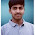### The Current Component of a p-n Junction Diode

It is indicated earlier that when a p-n junction diode is forward biased a large forward current flows, which is mainly due to majority carriers. The depletion region near the junction is very very small, under forward biased biased condition. In forward biased condition holes get diffused into n-side from p-side while electrons get diffused into p-side from n-side. So on p-side, the current carried by electrons which is diffusion current due to minority carriers, decreases exponentially with respect to distance measured from the junction. This current due to electrons, on p-side which are minority carriers is denoted as Inp. Similarly holes from p-side diffuse into n-side carry current which decreases exponentially with respect to distance measured from the junction. This current due to holes on n-side, which are minority carriers is denoted as Ipn. If distance is denoted by x then,
Inp(x) = Current due to electrons in p-side as a function of x
Ipn(x) = Current due to holes in n-side as a function of x
At the junction i.e. at x = 0, electrons crossing from n-side to p-side constitute a current, Inp(0) in the same direction as holes crossing the junction from p-side to n-side constitute a current, Ipn(0).
Hence the current at the junction is the total conventional current I flowing through the circuit.
...               I = Ipn(0) + Inp(0)                                             ..................... (1)
Now Ipn(x) decreases on n-side as we move away from junction on n-side. Similarly Inp(x) decreases on p-side as we move away from junction on p-side.
But as the entire circuit is a series circuit, the total current must be maintained at, independent of x. This indicates that on p-side there exists one more current component which is due to holes on p-side which are the majority carriers. It is denoted by Ipp (x) and the addition of the two currents on p-side is total current I.
Ipp (x) = Current due to holes in p-side.
Similarly on n-side, there exists one more current component which is due to electrons o n-side, which are the majority carriers. It is denoted as Inn(x) and the addition of the two currents on n-side is total current I.
Inn(x) = Current due to electrons in n-side.
On p-side,     I = Ipp(x) + Inp(x)                                      ................ (2)
On n-side,      I = Inn(x) + Ipn(x)                                     .................(3)
These current components are plotted as a function of distance in the Fig. 1.Fig.1  Current components
The current Ipp decreases towards the junction, at the junction enters the n-side and becomes Ipn which further decreases exponentially. Similarly the current Inn decreases towards the junction, at the junction enters the p-side and becomes Inp which also further decreases exponentially.
Note : In forward bias condition, the current enters the p-side as a hole current and leaves the n-side as an electron current, of the same magnitude.
So sum of the currents carried by electrons and holes at any point inside the diode is always constant equal to total forward current I. But the proportion due to holes and electrons in constituting the current varies with the distance, from the junction.

1.is that diagram fig1 correct?

1.2.is that diagram fig1 correct?

1.yes it is correct

3.is that diagram fig1 correct?

4.How does Ipp decreases

5.Because density of holes decreases as we move towards junction.